• +91 9971497814
• info@interviewmaterial.com

# Chapter 2- बहुपद (Poly) Ex-2.3 Interview Questions Answers

### Related Subjects

Question 1 : x3 + 3x² + 3x + 1 को निम्नलिखित से भाग देने पर शेषफल ज्ञात कीजिए :
(i) x + 1
(ii) x –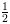(iii) x
(iv) x + π
(v) 5 + 2x

Answer 1 : माना p(x) = x3 + 3x² + 3x + 1
(i)
माना x+ 1 = 0 x = -1
p (x)
को +1 से भाग देने पर शेषफल
p(- 1) = (-1)3 + 3(-1)² + 3(-1) + 1 = -1 + 3 – 3 + 1 = 0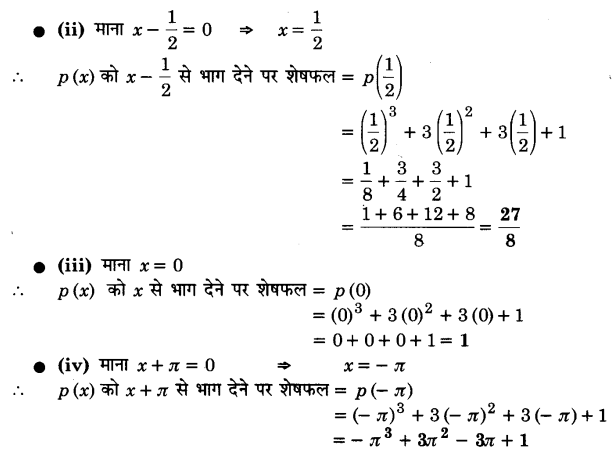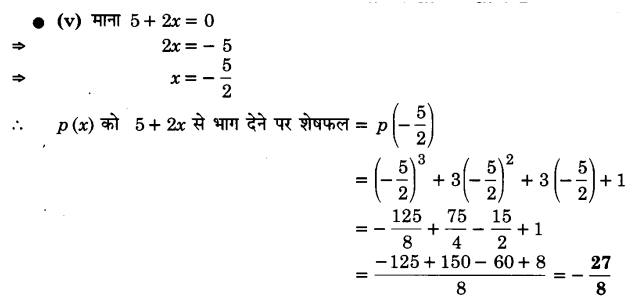Question 2 : x3 – ax² + 6x – a को x– a से भाग देने पर शेषफल ज्ञात कीजिए।

माना p (x) =x3 – ax² + 6x – a तथा x – a =0
p (x)
को x – a से भाग देने पर शेषफल = (a)3 – a(a)² + 6(a) – a = a3 –a3 + 6a – a = 5a

Question 3 : जाँच कीजिए कि 7+ 3x, 3x3 + 7x का एक गुणनखण्ड है या नहीं।

Answer 3 : माना p(x) = 3x + 7x
यदि 7+ 3x, p (x) का एक गुणनखण्ड है तो p(x) को 7+ 3x से भाग देने पर शेषफल शून्य होना चाहिए।
माना 7+ 3x = 0 3x= – 7 x =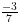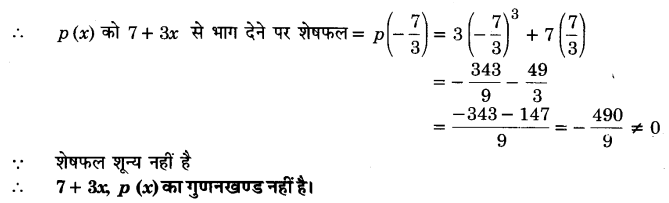Todays Deals### Chapter 2- बहुपद (Poly) Ex-2.3 Contributorskrishan

Name:
Email:

# Latest News# 9000 interview questions in different categories